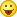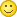# Need for New Efficiency Formula?

• I was thinking...with the elimination to Breeders and the addition of MOX fuel, does the old formula of neutron pulses divided by fuel rods still have a place? As far as I can tell, MOX fuel doesn't calculate its bonus based on neutron pulses...at least not directly. And it'd be nice to be able to see the efficiency of a MOX reactor at compared to a uranium one.

One way we can do this is by multiplying the MOX Bonus ((4*%ofmaxheat)+1) with the efficiency rating of a Uranium reactor using the same type of rods. This has the bonus of using game code (I couldn't find the source when looking the other day, but I believe Thunderdark posted it somewhere), and being fairly simple...but adds another number to the calculation that's dependent on a boolean (MOX Reactor = true/false).

Another way we could do it is by scrapping the whole neutron paradigm, and rewriting the formula used to calculate efficiency. And what is efficiency other than the power output per fuel rod? I'm sure if I get all mathematical and do the proofs, I can prove that the formula I'm about to suggest is the same formula as the old efficiency formula, just using other variables...but it's been awhile since I've been to math class, and math can be boring for some, so I'm not going to do that.

Anyways, I propose we calculate efficiency by dividing the power output (eu/t) by the rods used, and divide that by 20. If you'll look at my spreadsheet where I took my 14-rod MOX reactor, and all the reactors from the Official Reactor Design thread, the formula is completely backwards compatible with efficiency ratings using the old formula, but allows for accurate efficiency ratings for the new MOX reactors.

So what do you think? Good idea? Workable? Bad?

• So what do you think? Good idea? Workable? Bad?

I'm thinking, for the second time this week, that you really didn't read the MOX reactor thread at all, did youWe already went over this on page 1. Admittedly, back then things were a little bit more complicated, because you had to account for 1.) fuel rod efficiency from neutron pulses, 2.) heat efficiency from hull heat percentage, 3.) heat efficiency from absolute hull heat values, and 4.) bug efficiency from having the fuel rods as close as possible to the upper left corner of the setup. After Thunder's bugfixing spree (shortly before you came in), 3.) and 4.) stopped being relevant, so technically nowadays you just need to multiply your basic efficiency by the MOX heat multiplier and you're done. But the formula I proposed works just as well still, because it's completely agnostic of what multiplies where in what way. It works off of EU/t alone, which any player can see ingame in their reactor GUI without additional mods (or out of game tools) being required.

• What can I say...I'm very forgetful...